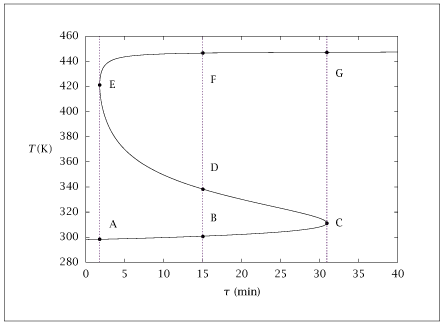## Figure 6.9:

### Steady-state temperature versus residence time for \Delta H_R = -3 x10^5kJ/kmol.## Code for Figure 6.9

### main.m

```
1
2
3
4
5
6
7
8
9
10
11
12
13
14
15
16
17
18
19
20
21
22
23
24
25
26
27
28
29
30
31
32
33
34
35
36
37
38
39
40
41
42
43
44
45
46
47
48
49
50
51
52
53
54
55
56
57
58
59
60
61
62
63
64
65
66
67
68
69
70
71
72
73
74
75
76% Copyright (C) 2001, James B. Rawlings and John G. Ekerdt
%
% This program is free software; you can redistribute it and/or
% modify it under the terms of the GNU General Public License as
% published by the Free Software Foundation; either version 2, or (at
% your option) any later version.
%
% This program is distributed in the hope that it will be useful, but
% WITHOUT ANY WARRANTY; without even the implied warranty of
% MERCHANTABILITY or FITNESS FOR A PARTICULAR PURPOSE.  See the GNU
% General Public License for more details.
%
% You should have received a copy of the GNU General Public License
% along with this program; see the file COPYING.  If not, write to
% the Free Software Foundation, 59 Temple Place - Suite 330, Boston,
% MA 02111-1307, USA.
%
% Revised 8/13/2018

%
% multiplicity parameters
%
% units are kmol, min, kJ, K, m^3
%
%
p = struct();
p.k_m      = 0.001;
p.T_m      = 298;
p.E        = 8000;
p.c_Af     = 2;
p.C_p      = 4;
p.rho      = 1000;
p.C_ps     = p.rho*p.C_p;
p.T_f      = 298;
p.T_a      = p.T_f;
p.U        = 0;
p.DeltaH_R = -3e5;
%
% limit cycle parameters
%
%p = struct();
%p.k_0      = 4.48e17;
%p.E        = 15000;
%p.c_Af     = 3;
%p.C_ps     = 4.19e3;
%p.T_f      = 298;
%p.T_a      = p.T_f;
%p.DeltaH_R = -1.571e5;
%p.U        = 6.029;
%p.theta    = 2085;

x0 = [1; p.T_f];
nc_As = 200;
tmp_table(1,:) = [0 p.T_f 0 0];
c_Avect = linspace(0.995*p.c_Af, .003*p.c_Af, nc_As);

for i = 1: nc_As
c_A = c_Avect(i);
opts = optimset ('MaxFunEvals', 2000*numel(x0), ...
'MaxIter', 500*numel(x0));
[x, fval, info] = fsolve(@(x) st_st_cA(x, c_A, p), x0, opts);
theta = x(1);
T     = x(2);
conv  = (p.c_Af - c_A)/p.c_Af;
tmp_table(i+1,:) = [theta, T, conv, info];
x0 = x;
end

table = [tmp_table];
save -ascii vanheerden_0.dat table;

if (~ strcmp (getenv ('OMIT_PLOTS'), 'true')) % PLOTTING
plot (table(:,1),table(:,2));
% TITLE
end % PLOTTING

```

### st_st_cA.m

```
1
2
3
4
5
6
7function retval = st_st_cA(x, c_A, p)
theta = x(1);
T     = x(2);
k     = p.k_m*exp(-p.E*(1/T - 1/p.T_m));
retval(1) = p.c_Af - (1 + k*theta)*c_A;
retval(2) = p.U*theta*(p.T_a - T) + p.C_ps*(p.T_f - T) -...
k*theta*c_A*p.DeltaH_R;

```